https://doi.org/10.5194/mr-2-395-2021
Special issue:
https://doi.org/10.5194/mr-2-395-2021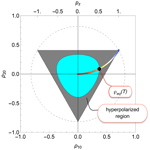# Hyperpolarization and the physical boundary of Liouville space

Malcolm H. Levitt and Christian Bengs
Abstract

The quantum state of a spin ensemble is described by a density operator, which corresponds to a point in the Liouville space of orthogonal spin operators. Valid density operators are confined to a particular region of Liouville space, which we call the physical region and which is bounded by multidimensional figures called simplexes. Each vertex of a simplex corresponds to a pure-state density operator. We provide examples for spins $I=\mathrm{1}/\mathrm{2}$, I=1, $I=\mathrm{3}/\mathrm{2}$ and for coupled pairs of spins-1/2. We use the von Neumann entropy as a criterion for hyperpolarization. It is shown that the inhomogeneous master equation for spin dynamics leads to non-physical results in some cases, a problem that may be avoided by using the Lindbladian master equation.

Share
Dates
1 Introduction

The central object of interest in nuclear magnetic resonance (NMR) theory is the density operator, which describes the quantum state of the ensemble of spin systems. It is defined as follows:

$\begin{array}{}\text{(1)}& \mathit{\rho }=\stackrel{\mathrm{‾}}{\mathrm{|}\mathit{\psi }〉〈\mathit{\psi }\mathrm{|}}.\end{array}$

Here |ψ specifies the quantum state of each individual spin system, and the overbar indicates an ensemble average . When expressed as a matrix in the eigenbasis of the coherent spin Hamiltonian, the diagonal elements are the spin state populations, and the off-diagonal elements are the coherences between the spin states.

It is often useful to express the density operator as a superposition of orthogonal spin operators. For example, the highly influential papers by Sørensen, Bodenhausen, Ernst and co-workers advocate an expansion in terms of Cartesian product operators , while some other groups favour spherical tensor operators . In all cases, the density operator is written in the form

$\begin{array}{}\text{(2)}& \mathit{\rho }=\sum _{q=\mathrm{1}}^{{N}_{L}}{\mathit{\rho }}_{q}{Q}_{q},\end{array}$

where the coefficients ρq are complex numbers in general, and the basis operators Qq are orthogonal:

$\begin{array}{}\text{(3)}& \left({Q}_{q}\mathrm{|}{Q}_{{q}^{\prime }}\right)=\text{Tr}\mathit{\left\{}{Q}_{q}^{†}{Q}_{{q}^{\prime }}\mathit{\right\}}={\mathit{\delta }}_{q{q}^{\prime }}‖{Q}_{q}{‖}^{\mathrm{2}}.\end{array}$

The Kronecker delta symbol δab takes the value 1 for a=b and 0 otherwise. The norm of the operator Qq is defined as $‖{Q}_{q}‖=\text{Tr}\mathit{\left\{}{Q}_{q}^{†}{{Q}_{q}}^{\mathrm{1}/\mathrm{2}}\mathit{\right\}}$.

The coefficients ρq are often given evocative names which suggest their physical interpretation, for example “antiphase order”, “zz order”, “spin alignment”, “Zeeman polarization”, ”singlet order”, and so on.

Since such expansions are nearly universal in modern NMR theory, it seems natural to pose questions of the forms “what values may the coefficients ρq take?”, “are the values of ρq unlimited, or bounded in some way?”, “does the value of one coefficient influence the possible values of a second coefficient?”, etc. Surprisingly, these natural questions are rarely posed in the NMR world, although they have not escaped the attention of mathematical physicists and applied mathematicians .

The expansion in Eq. (2) identifies the density operator with a point in a multidimensional space with coordinates {ρ1,ρ2}. This space has been called Liouville space . In this article, we show that valid density operators may only be identified with points in a defined region of Liouville space which we call the physical region. The physical region is enclosed by a convex boundary, which we call the physical boundary of Liouville space. We ask: what is the shape of the physical boundary? Does it have straight edges, or is it spherical in all dimensions? (Spoiler: at least some edges are straight.)

In addition, we express some views on the nature and definition of hyperpolarization. For example, is pure parahydrogen hyperpolarized, even though it generates no NMR signal? (Spoiler: our answer is yes.)

2 Orthonormal operators

To facilitate the discussion, the basis operators Qq in Eq. (2) are henceforth considered to be normalized as well as orthogonal, so that Eq. (3) is replaced by the simpler form

$\begin{array}{}\text{(4)}& \left({Q}_{q}\mathrm{|}{Q}_{{q}^{\prime }}\right)=\text{Tr}\mathit{\left\{}{Q}_{q}^{†}{Q}_{{q}^{\prime }}\mathit{\right\}}={\mathit{\delta }}_{q{q}^{\prime }}.\end{array}$

Note, however, that the Cartesian product operators advocated by are not normalized.

In general, NL operators are required in the expansion of Eq. (2), where ${N}_{L}={N}_{H}^{\mathrm{2}}$ and NH is the dimension of the Hilbert space of the individual spin systems. The orthonormal operators $\mathit{\left\{}{Q}_{\mathrm{1}},{Q}_{\mathrm{2}}\mathrm{\dots }{Q}_{{N}_{L}}\mathit{\right\}}$ define a NL-dimensional Liouville space . The density operator may be represented as a point with coordinates $\mathit{\left\{}{q}_{\mathrm{1}},{q}_{\mathrm{2}}\mathrm{\dots }{q}_{{N}_{L}}\mathit{\right\}}$ in this space. All spin dynamics may be represented as a trajectory traced by the spin density operator as it moves through this abstract space.

Brief consideration shows that there must be limits to the physical region of Liouville space. Consider for example an ensemble of isolated spins-1/2. In this case, the dimension of Hilbert space is NH=2, and the dimension of Liouville space is NL=4. The following four normalized operators may be chosen as the basis of Liouville space:

$\begin{array}{}\text{(5)}& \begin{array}{rl}{Q}_{\mathrm{1}}={\mathrm{2}}^{-\mathrm{1}/\mathrm{2}}\mathbb{I}\phantom{\rule{1em}{0ex}}\phantom{\rule{1em}{0ex}}\phantom{\rule{1em}{0ex}}& {Q}_{\mathrm{2}}={\mathrm{2}}^{\mathrm{1}/\mathrm{2}}{I}_{x}\\ {Q}_{\mathrm{3}}={\mathrm{2}}^{\mathrm{1}/\mathrm{2}}{I}_{y}\phantom{\rule{1em}{0ex}}\phantom{\rule{1em}{0ex}}\phantom{\rule{1em}{0ex}}& {Q}_{\mathrm{4}}={\mathrm{2}}^{\mathrm{1}/\mathrm{2}}{I}_{z}\end{array}\end{array}$

Since Tr{ρ}=1 by definition, the first coefficient is fixed at ${q}_{\mathrm{1}}={\mathrm{2}}^{-\mathrm{1}/\mathrm{2}}$, the density operator is only free to move in the subspace formed by the other three operators, $\mathit{\left\{}{Q}_{\mathrm{2}},{Q}_{\mathrm{3}},{Q}_{\mathrm{4}}\mathit{\right\}}$, which are proportional to the angular momentum operators in the three Cartesian directions.

The populations of the two Zeeman states are given by

$\begin{array}{}\text{(6)}& \begin{array}{rl}〈\mathit{\alpha }\mathrm{|}\mathit{\rho }\mathrm{|}\mathit{\alpha }〉& ={\mathrm{2}}^{-\mathrm{1}/\mathrm{2}}\left({q}_{\mathrm{1}}+{q}_{\mathrm{4}}\right)\\ 〈\mathit{\beta }\mathrm{|}\mathit{\rho }\mathrm{|}\mathit{\beta }〉& ={\mathrm{2}}^{-\mathrm{1}/\mathrm{2}}\left({q}_{\mathrm{1}}-{q}_{\mathrm{4}}\right)\end{array}\end{array}$

Both state populations are, by definition, bounded by 0 and 1:

$\begin{array}{}\text{(7)}& \begin{array}{r}\mathrm{0}\le 〈\mathit{\alpha }\mathrm{|}\mathit{\rho }\mathrm{|}\mathit{\alpha }〉\le \mathrm{1}\\ \mathrm{0}\le 〈\mathit{\beta }\mathrm{|}\mathit{\rho }\mathrm{|}\mathit{\beta }〉\le \mathrm{1}\end{array}\end{array}$

Hence the coefficient q4 is bounded as follows:

$\begin{array}{}\text{(8)}& -{\mathrm{2}}^{-\mathrm{1}/\mathrm{2}}\le {q}_{\mathrm{4}}\le {\mathrm{2}}^{-\mathrm{1}/\mathrm{2}}.\end{array}$

The upper bound ${q}_{\mathrm{4}}={\mathrm{2}}^{-\mathrm{1}/\mathrm{2}}$ corresponds to maximum spin polarization along the positive z axis, with the |α state completely populated and the |β state completely depleted. The lower bound ${q}_{\mathrm{4}}=-{\mathrm{2}}^{-\mathrm{1}/\mathrm{2}}$ corresponds to maximum spin polarization along the negative z axis, with the |α state completely depleted and the |β state completely populated.

In the case of isolated spins-1/2, the physical bounds on Liouville space are therefore defined by the fixing of one coordinate (${q}_{\mathrm{1}}={\mathrm{2}}^{-\mathrm{1}/\mathrm{2}}$) and the constraint of the other three to the interior of a sphere of radius ${\mathrm{2}}^{-\mathrm{1}/\mathrm{2}}$. Within a numerical factor, this geometrical bound is of course identical to the familiar Bloch sphere – the seminal geometrical object in magnetic resonance theory.

What about systems other than spins-1/2? Liouville space has more than three dimensions in such cases and is hard to visualize. Nevertheless, it is tempting to assume that the physical bounds are still spherical, albeit with an extension to higher dimensions. However, this turns out to be incorrect, in general. The physical bounds in some of the dimensions of Liouville space turn out not to be spheres but regular simplexes. A regular simplex in one dimension is a line, a regular simplex in two dimensions is an equilateral triangle, and a regular simplex in three dimensions is a regular tetrahedron, with the concept extending to arbitrary dimensions. In general, a simplex is the simplest possible convex object, where the term convex means that any two points belonging to the object may be connected by a straight line which never leaves the object. In general, a simplex in N dimensions is called an N-simplex, although some simplexes also have special names, such as the line (1-simplex), the triangle (2-simplex), the tetrahedron (3-simplex), and the pentachoron or 5-cell (4-simplex) (Coxeter1963).

The physical boundary of Liouville space is of little consequence for “conventional” NMR experiments, which are performed at or near thermal equilibrium. Under ordinary temperatures and magnetic fields, this is a region very close to the origin of Liouville space (except for the fixed projection onto the unity operator) and hence very far from the boundary. However, hyperpolarization techniques such as optical pumping , dynamic nuclear polarization , quantum-rotor-induced polarization and parahydrogen-induced polarization have provided ready access to regions which are “close to the edge”. Furthermore, spin systems which are in a highly non-equilibrium state are of great practical importance because of the greatly enhanced NMR signals that they can produce. The position and shape of the physical boundary have therefore become relevant.

Furthermore, some familiar concepts in magnetic resonance which were originally developed in the context of near-equilibrium spin dynamics do not retain validity far from the origin. An important case is the inhomogeneous master equation , which fails close to the physical boundary of Liouville space, where it should be replaced by a Lindbladian master equation .

3 Polarization moments

## 3.1 Isolated spins-I

For an ensemble of isolated spins-I, a suitable expansion of the form in Eq. (2) is as follows:

$\begin{array}{}\text{(9)}& \mathit{\rho }=\sum _{\mathit{\lambda }=\mathrm{0}}^{\mathrm{2}I}\phantom{\rule{0.33em}{0ex}}\sum _{\mathit{\mu }=-I}^{+I}{\mathit{\rho }}_{\mathit{\lambda }\mathit{\mu }}{\mathbb{T}}_{\mathit{\lambda }\mathit{\mu }}.\end{array}$

Here ρλμ are complex numbers which are called here polarization moments, following the usage in the atomic physics community . The operators 𝕋λμ are normalized irreducible spherical tensor operators (NISTOs). They are normalized over the spin-I Hilbert space:

$\begin{array}{}\text{(10)}& \left({\mathbb{T}}_{\mathit{\lambda }\mathit{\mu }}\mathrm{|}{\mathbb{T}}_{\mathit{\lambda }\mathit{\mu }}\right)=\text{Tr}\mathit{\left\{}{\mathbb{T}}_{\mathit{\lambda }\mathit{\mu }}^{{}^{†}}{\mathbb{T}}_{\mathit{\lambda }\mathit{\mu }}\mathit{\right\}}=\mathrm{1}.\end{array}$

The normalized spherical tensor operators 𝕋λμ differ from the operators Tλμ commonly used in NMR theory by a multiplicative factor.

A semantic objection may be raised over the use of the term polarization moment for the case λ=0. The term polarization is generally taken to imply an anisotropic distribution of dipole moments (magnetic or electric). However, the rank-0 moment represents an isotropic distribution of spin angular momentum and hence is not a “polarization” in a conventional sense. While acknowledging that this is a reasonable objection, we contend that the extension of the term “polarization” to cover rank-0 terms is too convenient to be blocked by pedantry. A similar objection arises over the term singlet polarization for spin pairs, as discussed below.

For isolated spins-I, the low-rank normalized spherical tensor operators are as follows, for the case μ=0:

$\begin{array}{}\text{(11)}& \begin{array}{rl}{\mathbb{T}}_{\mathrm{00}}& ={\mathbb{N}}_{\mathrm{0}}^{I}\mathbb{I}\\ {\mathbb{T}}_{\mathrm{10}}& ={\mathbb{N}}_{\mathrm{1}}^{I}{I}_{z}\\ {\mathbb{T}}_{\mathrm{20}}& ={\mathbb{N}}_{\mathrm{2}}^{I}\frac{\mathrm{1}}{\sqrt{\mathrm{6}}}\left(\mathrm{3}{{I}_{z}}^{\mathrm{2}}-I\left(I+\mathrm{1}\right)\mathbb{I}\right)\\ {\mathbb{T}}_{\mathrm{30}}& ={\mathbb{N}}_{\mathrm{3}}^{I}\frac{\mathrm{1}}{\sqrt{\mathrm{10}}}\left(\mathrm{5}{{I}_{z}}^{\mathrm{3}}+\left(\mathrm{1}-\mathrm{3}I\left(I+\mathrm{1}\right)\right){I}_{z}\right)\end{array}\end{array}$

The normalization factors are as follows:

$\begin{array}{}\text{(12)}& \begin{array}{rl}{\mathbb{N}}_{\mathrm{0}}^{I}& =\left(\mathrm{2}I+\mathrm{1}{\right)}^{-\mathrm{1}/\mathrm{2}}\\ {\mathbb{N}}_{\mathrm{1}}^{I}& =\mathit{\left\{}\frac{\mathrm{1}}{\mathrm{3}}I\left(I+\mathrm{1}\right)\left(\mathrm{2}I+\mathrm{1}\right){\mathit{\right\}}}^{-\mathrm{1}/\mathrm{2}}\\ {\mathbb{N}}_{\mathrm{2}}^{I}& =\mathit{\left\{}\frac{\mathrm{1}}{\mathrm{30}}I\left(I+\mathrm{1}\right)\left(\mathrm{2}I-\mathrm{1}\right)\left(\mathrm{2}I+\mathrm{3}\right)\left(\mathrm{2}I+\mathrm{1}\right){\mathit{\right\}}}^{-\mathrm{1}/\mathrm{2}}\\ {\mathbb{N}}_{\mathrm{3}}^{I}& =\mathit{\left\{}\frac{\mathrm{1}}{\mathrm{70}}I\left(I+\mathrm{1}\right)\left(I-\mathrm{1}\right)\left(I+\mathrm{2}\right)\left(\mathrm{2}I-\mathrm{1}\right)\\ & \phantom{\rule{0.25em}{0ex}}\phantom{\rule{0.25em}{0ex}}×\left(\mathrm{2}I+\mathrm{3}\right)\left(\mathrm{2}I+\mathrm{1}\right){\mathit{\right\}}}^{-\mathrm{1}/\mathrm{2}}\end{array}\end{array}$

These normalization factors depend on the spin quantum number I and the rank λ but are independent of the component index μ.

It follows from Eqs. (2) and (10) and the orthogonality of the NISTOs that any polarization moment may be derived from the density operator by a Liouville bracket operation:

$\begin{array}{}\text{(13)}& {\mathit{\rho }}_{\mathit{\lambda }\mathit{\mu }}=\left({\mathbb{T}}_{\mathit{\lambda }\mathit{\mu }}\mathrm{|}\mathit{\rho }\right)=\text{Tr}\mathit{\left\{}{\mathbb{T}}_{\mathit{\lambda }\mathit{\mu }}^{{}^{†}}\mathit{\rho }\mathit{\right\}}.\end{array}$

The polarization moments have the following symmetry:

$\begin{array}{}\text{(14)}& {\mathit{\rho }}_{\mathit{\lambda }\mathit{\mu }}=\left(-\mathrm{1}{\right)}^{-\mathit{\mu }}{\mathit{\rho }}_{\mathit{\lambda }-\mathit{\mu }}^{*},\end{array}$

which follows from the hermiticity of the density operator and the symmetries of the spherical tensor operator components .

For isolated spins-I, the condition Tr{ρ}=1 fixes the value of the rank-0 polarization moment:

$\begin{array}{}\text{(15)}& {\mathit{\rho }}_{\mathrm{00}}=\left(\mathrm{2}I+\mathrm{1}{\right)}^{-\mathrm{1}/\mathrm{2}}.\end{array}$

The rank-1 polarization moment ρ10 is proportional to the z polarization of the spin-I ensemble as follows:

$\begin{array}{}\text{(16)}& {\mathit{\rho }}_{\mathrm{10}}=\mathit{\left\{}\frac{\mathrm{1}}{\mathrm{3}I}\left(I+\mathrm{1}\right)\left(\mathrm{2}I+\mathrm{1}\right){\mathit{\right\}}}^{-\mathrm{1}/\mathrm{2}}\phantom{\rule{0.33em}{0ex}}{p}_{z}.\end{array}$

Similarly, the rank-1 polarization moments ρ1±1 are proportional to complex combinations of the transverse polarizations:

$\begin{array}{}\text{(17)}& {\mathit{\rho }}_{\mathrm{1}±\mathrm{1}}=\mathit{\left\{}\frac{\mathrm{2}}{\mathrm{3}I}\left(I+\mathrm{1}\right)\left(\mathrm{2}I+\mathrm{1}\right){\mathit{\right\}}}^{-\mathrm{1}/\mathrm{2}}\left(\mp {p}_{x}+i{p}_{y}\right).\end{array}$

The relationship in Eq. (16) evaluates as follows for some common spin quantum numbers I:

$\begin{array}{}\text{(18)}& \begin{array}{rl}{\mathit{\rho }}_{\mathrm{10}}={\mathrm{2}}^{-\mathrm{1}/\mathrm{2}}\phantom{\rule{0.33em}{0ex}}{p}_{z}\phantom{\rule{1em}{0ex}}\phantom{\rule{1em}{0ex}}& \text{(}I=\mathrm{1}/\mathrm{2}\text{)}\\ {\mathit{\rho }}_{\mathrm{10}}={\mathrm{2}}^{-\mathrm{1}/\mathrm{2}}\phantom{\rule{0.33em}{0ex}}{p}_{z}\phantom{\rule{1em}{0ex}}\phantom{\rule{1em}{0ex}}& \text{(}I=\mathrm{1}\text{)}\\ {\mathit{\rho }}_{\mathrm{10}}=\frac{\mathrm{3}}{\mathrm{2}}{\mathrm{5}}^{-\mathrm{1}/\mathrm{2}}\phantom{\rule{0.33em}{0ex}}{p}_{z}\phantom{\rule{1em}{0ex}}\phantom{\rule{1em}{0ex}}& \text{(}I=\mathrm{3}/\mathrm{2}\text{)}\\ {\mathit{\rho }}_{\mathrm{10}}=\left(\frac{\mathrm{5}}{\mathrm{14}}{\right)}^{\mathrm{1}/\mathrm{2}}\phantom{\rule{0.33em}{0ex}}{p}_{z}\phantom{\rule{1em}{0ex}}\phantom{\rule{1em}{0ex}}& \text{(}I=\mathrm{5}/\mathrm{2}\text{)}\end{array}\end{array}$

In atomic physics, finite moments with rank λ=1 are called orientation, while finite moments with rank λ=2 are called alignment . Although the term orientation is not generally used for this purpose in the magnetic resonance community, the term alignment is used to imply rank-2 multipole order, particularly in the context of solid-state NMR as applied to quadrupolar nuclei . For isolated spins-I, the terms spin alignment and quadrupolar order may be regarded as synonymous.

Multipole expansions of the spin density operator as in Eq. (2) have long been used in NMR. Extensive theoretical development was performed by Sanctuary, Bowden, Bain and co-workers and has been exploited to generate graphical representations of density operator evolution . One of the salient early examples of the multipole description is the treatment of quadrupolar relaxation by Bodenhausen and co-workers . In this elegant paper, the relaxation dynamics of quadrupolar nuclei outside the extreme narrowing limit is treated in terms of propagation in the space of spherical tensor operators, drawing fruitful parallels with the concepts of coherence transfer pathways .

There are also techniques for determining the polarization moments of a spin ensemble experimentally at any point during a pulse sequence by combining the signals from many successive experiments multiplied by complex factors. This method has been called spherical tensor analysis and has been applied to the study of endofullerenes .

## 3.2 Spin-1/2 pairs

The construction of spherical tensor operators for systems of coupled spins is a complicated affair. Extensive expositions of the technique have been given . In this article, the discussion of coupled spin systems is restricted to the simplest case, namely pairs of coupled spins-1/2. Since the dimension of Hilbert space is NH=4, the dimension of Liouville space is NL=16. This space includes six orthogonal zero-quantum operators, four of which are symmetric with respect to spin exchange and two of which are antisymmetric. The four symmetric μ=0 operators are as follows:

$\begin{array}{}\text{(19)}& \begin{array}{rl}{\mathbb{T}}_{\mathrm{00}}^{\mathrm{0}}& =\frac{\mathrm{1}}{\mathrm{2}}\mathbb{I},\\ {\mathbb{T}}_{\mathrm{00}}^{\mathrm{12}}& =-\mathrm{2}×{\mathrm{3}}^{-\mathrm{1}/\mathrm{2}}{\mathbf{I}}_{\mathrm{1}}\cdot {\mathbf{I}}_{\mathrm{2}},\\ {\mathbb{T}}_{\mathrm{10}}^{+}& ={\mathrm{2}}^{-\mathrm{1}/\mathrm{2}}\left({I}_{\mathrm{1}z}+{I}_{\mathrm{2}z}\right),\\ {\mathbb{T}}_{\mathrm{20}}^{\mathrm{12}}& =\left(\mathrm{2}/\mathrm{3}{\right)}^{\mathrm{1}/\mathrm{2}}\left(\mathrm{3}{I}_{\mathrm{1}z}{I}_{\mathrm{2}z}-{\mathbf{I}}_{\mathrm{1}}\cdot {\mathbf{I}}_{\mathrm{2}}\right).\end{array}\end{array}$

Note that spin-1/2 pairs support two different spherical tensor operators with rank λ=0, denoted ${\mathbb{T}}_{\mathrm{00}}^{\mathrm{0}}$ and ${\mathbb{T}}_{\mathrm{00}}^{\mathrm{12}}$. The plus superscript in ${\mathbb{T}}_{\mathrm{10}}^{+}$ indicates that the operators I1z and I2z are combined with the same sign.

The operator ${\mathbb{T}}_{\mathrm{00}}^{\mathrm{0}}$ is proportional to the unity operator. The corresponding polarization moment is fixed by the condition Tr{ρ}=1 to the value

$\begin{array}{}\text{(20)}& {\mathit{\rho }}_{\mathrm{00}}^{\mathrm{0}}=\frac{\mathrm{1}}{\mathrm{2}}.\end{array}$

The operator ${\mathbb{T}}_{\mathrm{00}}^{\mathrm{12}}$ is proportional to the scalar product of the two spin angular momenta. The corresponding polarization moment is given by

$\begin{array}{}\text{(21)}& {\mathit{\rho }}_{\mathrm{00}}^{\mathrm{12}}=\frac{\sqrt{\mathrm{3}}}{\mathrm{2}}{p}_{\mathrm{S}},\end{array}$

where pS is called the singlet polarization or singlet order and corresponds to the population imbalance between the singlet state and the triplet manifold, in the spin-pair ensemble. In many cases, the singlet polarization is protected against common relaxation mechanisms and exhibits an extended lifetime .

Since the moments ${\mathit{\rho }}_{\mathrm{00}}^{\mathrm{0}}$ and ${\mathit{\rho }}_{\mathrm{00}}^{\mathrm{12}}$ have spherical rank λ=0, they both represent isotropic distributions of the spin angular momenta. As before, we contend that the extension of the term “polarization” to cover rank-0 spherical moments is too convenient to ignore while accepting that opinions may differ on the wisdom of this approach.

The operator ${\mathbb{T}}_{\mathrm{10}}^{+}$ corresponds to a symmetric combination of the z-angular momentum operators for the two spins. The corresponding polarization moment is proportional to the mean z polarization of the spin ensemble:

$\begin{array}{}\text{(22)}& {\mathit{\rho }}_{\mathrm{10}}^{+}=\frac{\mathrm{1}}{\sqrt{\mathrm{2}}}{p}_{z}.\end{array}$

The operator ${\mathbb{T}}_{\mathrm{20}}^{\mathrm{12}}$ corresponds to the rank-2 spherical tensor operator of the coupled spin pair. The corresponding polarization moment ${\mathit{\rho }}_{\mathrm{20}}^{\mathrm{12}}$ is proportional to the rank-2 order (dipolar order) of the spin-pair ensemble.

4 Physical bounds of Liouville space

The population of each individual spin state is bounded by 0 and 1, while the sum of all populations is equal to 1. These properties constrain the physically realizable values of the polarization moments.

The spin density operator of the isolated spin-I ensemble may be expressed as a superposition of spherical tensor operators, with rank λ taking values between 0 and 2I. The rank-0 moment is fixed to the value ${\mathit{\rho }}_{\mathrm{00}}=\left(\mathrm{2}I+\mathrm{1}{\right)}^{-\mathrm{1}}$ (Eq. 15). From Eq. (2) and the orthonormality of the NISTOs (Eq. 10), the population of the state |I,MI may be written as follows:

$\begin{array}{}\text{(23)}& 〈I,{M}_{I}\mathrm{|}\mathit{\rho }\mathrm{|}I,{M}_{I}〉=\sum _{\mathit{\lambda }=\mathrm{0}}^{\mathrm{2}I}〈I,{M}_{I}\mathrm{|}{\mathbb{T}}_{\mathit{\lambda }\mathrm{0}}\mathrm{|}I,{M}_{I}〉{\mathit{\rho }}_{\mathit{\lambda }\mathrm{0}}.\end{array}$

Hence, for isolated spins-I, there exists a system of 2I+1 simultaneous inequalities on the μ=0 polarization moments:

$\begin{array}{}\text{(24)}& \mathrm{0}\phantom{\rule{0.33em}{0ex}}\le \phantom{\rule{0.33em}{0ex}}\sum _{\mathit{\lambda }=\mathrm{0}}^{\mathrm{2}I}\phantom{\rule{0.33em}{0ex}}〈I,{M}_{I}\mathrm{|}{\mathbb{T}}_{\mathit{\lambda }\mathrm{0}}\mathrm{|}I,{M}_{I}〉{\mathit{\rho }}_{\mathit{\lambda }\mathrm{0}}\phantom{\rule{0.33em}{0ex}}\le \phantom{\rule{0.33em}{0ex}}\mathrm{1},\end{array}$

for ${M}_{I}\in \mathit{\left\{}+I,+I-\mathrm{1}\mathrm{\dots }-I\mathit{\right\}}$. Together with Eq. (15), the system of inequalities in Eq. (24) defines the physical bounds of the μ=0 polarization moments.

The consequences are now explored for some common spin systems.

## 4.1 Spins-1/2

For isolated spins-1/2, the rank-0 polarization moment is given from Eq. (15) by

$\begin{array}{}\text{(25)}& {\mathit{\rho }}_{\mathrm{00}}={\mathrm{2}}^{-\mathrm{1}/\mathrm{2}}\phantom{\rule{1em}{0ex}}\phantom{\rule{1em}{0ex}}\text{for}\phantom{\rule{0.25em}{0ex}}I=\mathrm{1}/\mathrm{2}.\end{array}$

Equations (24) and (25) lead to the following physical bounds for the rank-1 polarization moment:

$\begin{array}{}\text{(26)}& -{\mathrm{2}}^{-\mathrm{1}/\mathrm{2}}\phantom{\rule{0.33em}{0ex}}\le \phantom{\rule{0.33em}{0ex}}{\mathit{\rho }}_{\mathrm{10}}\phantom{\rule{0.33em}{0ex}}\le \phantom{\rule{0.33em}{0ex}}+{\mathrm{2}}^{-\mathrm{1}/\mathrm{2}}\phantom{\rule{1em}{0ex}}\phantom{\rule{1em}{0ex}}\text{for}\phantom{\rule{0.25em}{0ex}}I=\mathrm{1}/\mathrm{2}.\end{array}$

From Eq. (16), this corresponds to the expected bounds on the z polarization of the spin ensemble:

$\begin{array}{}\text{(27)}& -\mathrm{1}\phantom{\rule{0.33em}{0ex}}\le \phantom{\rule{0.33em}{0ex}}{p}_{z}\phantom{\rule{0.33em}{0ex}}\le \phantom{\rule{0.33em}{0ex}}+\mathrm{1},\end{array}$

which should come as no surprise. No spin system may have more than 100 % polarization.

## 4.2 Spins-1

The bounds on the polarization moments are more complicated for an ensemble of isolated spins-1. The rank-0 polarization moment is given through Eq. (15) by

$\begin{array}{}\text{(28)}& {\mathit{\rho }}_{\mathrm{00}}={\mathrm{3}}^{-\mathrm{1}/\mathrm{2}}\phantom{\rule{1em}{0ex}}\phantom{\rule{1em}{0ex}}\text{for}\phantom{\rule{0.25em}{0ex}}I=\mathrm{1}.\end{array}$

The inequalities on the rank-1 and rank-2 polarization moments evaluate as follows:

$\begin{array}{}\text{(29)}& \begin{array}{rl}\mathrm{0}\phantom{\rule{0.33em}{0ex}}\le & \frac{\mathrm{1}}{\mathrm{3}}+\frac{\mathrm{1}}{\sqrt{\mathrm{2}}}{\mathit{\rho }}_{\mathrm{10}}+\frac{\mathrm{1}}{\sqrt{\mathrm{6}}}{\mathit{\rho }}_{\mathrm{20}}\phantom{\rule{0.33em}{0ex}}\le \phantom{\rule{0.33em}{0ex}}\mathrm{1},\\ \mathrm{0}\phantom{\rule{0.33em}{0ex}}\le & \frac{\mathrm{1}}{\mathrm{3}}-\sqrt{\frac{\mathrm{2}}{\mathrm{3}}}{\mathit{\rho }}_{\mathrm{20}}\phantom{\rule{0.33em}{0ex}}\le \phantom{\rule{0.33em}{0ex}}\mathrm{1},\\ \mathrm{0}\phantom{\rule{0.33em}{0ex}}\le & \frac{\mathrm{1}}{\mathrm{3}}-\frac{\mathrm{1}}{\sqrt{\mathrm{2}}}{\mathit{\rho }}_{\mathrm{10}}+\frac{\mathrm{1}}{\sqrt{\mathrm{6}}}{\mathit{\rho }}_{\mathrm{20}}\phantom{\rule{0.33em}{0ex}}\le \phantom{\rule{0.33em}{0ex}}\mathrm{1}\phantom{\rule{1em}{0ex}}\phantom{\rule{1em}{0ex}}\text{for}\phantom{\rule{0.25em}{0ex}}I=\mathrm{1}.\end{array}\end{array}$

The values of {ρ10,ρ20} which satisfy the inequalities in Eq. (29) lie within the shaded triangle in Fig. 1. The vertices of the triangle have coordinates {ρ10,ρ20} given by $\mathit{\left\{}±{\mathrm{2}}^{-\mathrm{1}/\mathrm{2}},{\mathrm{6}}^{-\mathrm{1}/\mathrm{2}}\mathit{\right\}}$ and $\mathit{\left\{}\mathrm{0},-\left(\mathrm{2}/\mathrm{3}{\right)}^{\mathrm{1}/\mathrm{2}}\mathit{\right\}}$; each vertex corresponds to a pure-state density operator, in which only one state is populated. Similar triangular bounds have been identified in the mathematics literature .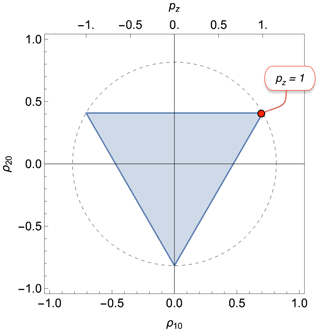Figure 1Physical bounds on the rank-1 and rank-2 polarization moments for isolated spins I=1. The shaded triangle shows the physically accessible region. The vertices correspond to pure-state density operators for each of the three spin-1 Zeeman states. The radius of the dashed circle is $\sqrt{\mathrm{2}/\mathrm{3}}$. The rank-1 polarization moment ρ10 is related to the z polarization pz through Eq. (18). The red circle indicates a state with maximum Zeeman polarization.

The equilateral triangle in Fig. 1 corresponds to a regular simplex in two dimensions.

The maximum z polarization of pz=1 corresponds to the upper-right vertex. Figure 1 shows that this highly polarized state corresponds to a mixture of rank-1 polarization (Zeeman order) and rank-2 polarization (quadrupolar order). It follows that the near-complete hyperpolarization of spin-1 nuclei, as performed by the Bodenhausen group , generates hyperpolarized quadrupolar order as well as Zeeman order.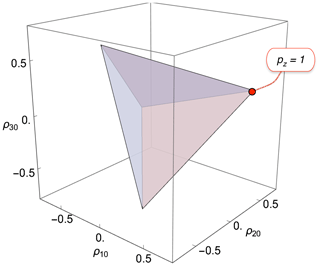Figure 2Physical bounds on the rank-1, rank-2, rank-3 polarization moments for isolated spins $I=\mathrm{3}/\mathrm{2}$. The shaded tetrahedron shows the physically accessible region. The vertices correspond to pure-state density operators for each of the four spin-1 Zeeman states. The red circle indicates the position of maximum Zeeman polarization.

## 4.3 Spins-3/2

In the case of isolated spins-3/2, the rank-0 polarization moment is given by

$\begin{array}{}\text{(30)}& {\mathit{\rho }}_{\mathrm{00}}={\mathrm{4}}^{-\mathrm{1}/\mathrm{2}}=\mathrm{1}/\mathrm{2}\phantom{\rule{1em}{0ex}}\phantom{\rule{1em}{0ex}}\text{for}\phantom{\rule{0.25em}{0ex}}I=\mathrm{3}/\mathrm{2}.\end{array}$

For spins-3/2, there may be a finite rank-3 polarization moment ρ30 as well as the rank-1 and rank-2 terms. The physical bounds on these polarization moments are set by the following inequalities:

$\begin{array}{}\text{(31)}& \begin{array}{rl}\mathrm{0}\phantom{\rule{0.33em}{0ex}}\le & \frac{\mathrm{1}}{\mathrm{20}}\left(\mathrm{5}+\mathrm{6}\sqrt{\mathrm{5}}{\mathit{\rho }}_{\mathrm{10}}+\mathrm{10}{\mathit{\rho }}_{\mathrm{20}}+\mathrm{2}\sqrt{\mathrm{5}}{\mathit{\rho }}_{\mathrm{30}}\right)\phantom{\rule{0.33em}{0ex}}\le \phantom{\rule{0.33em}{0ex}}\mathrm{1}\\ \mathrm{0}\phantom{\rule{0.33em}{0ex}}\le & \frac{\mathrm{1}}{\mathrm{20}}\left(\mathrm{5}+\mathrm{2}\sqrt{\mathrm{5}}{\mathit{\rho }}_{\mathrm{10}}-\mathrm{10}{\mathit{\rho }}_{\mathrm{20}}-\mathrm{6}\sqrt{\mathrm{5}}{\mathit{\rho }}_{\mathrm{30}}\right)\phantom{\rule{0.33em}{0ex}}\le \phantom{\rule{0.33em}{0ex}}\mathrm{1}\\ \mathrm{0}\phantom{\rule{0.33em}{0ex}}\le & \frac{\mathrm{1}}{\mathrm{20}}\left(\mathrm{5}-\mathrm{2}\sqrt{\mathrm{5}}{\mathit{\rho }}_{\mathrm{10}}-\mathrm{10}{\mathit{\rho }}_{\mathrm{20}}+\mathrm{6}\sqrt{\mathrm{5}}{\mathit{\rho }}_{\mathrm{30}}\right)\phantom{\rule{0.33em}{0ex}}\le \phantom{\rule{0.33em}{0ex}}\mathrm{1}\\ \mathrm{0}\phantom{\rule{0.33em}{0ex}}\le & \frac{\mathrm{1}}{\mathrm{20}}\left(\mathrm{5}-\mathrm{6}\sqrt{\mathrm{5}}{\mathit{\rho }}_{\mathrm{10}}+\mathrm{10}{\mathit{\rho }}_{\mathrm{20}}-\mathrm{2}\sqrt{\mathrm{5}}{\mathit{\rho }}_{\mathrm{30}}\right)\phantom{\rule{0.33em}{0ex}}\le \phantom{\rule{0.33em}{0ex}}\mathrm{1}\\ & \phantom{\rule{0.25em}{0ex}}\phantom{\rule{0.25em}{0ex}}\text{for}\phantom{\rule{0.25em}{0ex}}\phantom{\rule{0.25em}{0ex}}I=\mathrm{3}/\mathrm{2}.\end{array}\end{array}$

The physical bounds on the three polarization moments constrain the spin density operator to the interior of the regular tetrahedron shown in Fig. 2. The vertices of the tetrahedron are at coordinates $\mathit{\left\{}{\mathit{\rho }}_{\mathrm{10}},{\mathit{\rho }}_{\mathrm{20}},{\mathit{\rho }}_{\mathrm{30}}\mathit{\right\}}$ given by $\mathit{\left\{}±\mathrm{1}/\mathrm{2}\sqrt{\mathrm{5}},-\mathrm{1}/\mathrm{2},\mp \mathrm{3}/\mathrm{2}\sqrt{\mathrm{5}}\mathit{\right\}}$ and $\mathit{\left\{}±\mathrm{3}/\mathrm{2}\sqrt{\mathrm{5}},\mathrm{1}/\mathrm{2},±\mathrm{1}/\mathrm{2}\sqrt{\mathrm{5}}\mathit{\right\}}$. Each vertex corresponds to a pure-state density operator, in which only one state is populated. The z polarization is related to the rank-1 polarization moment ρ10 through Eq. (18).

The tetrahedron in Fig. 2 corresponds to a regular simplex in three dimensions.

## 4.4 Higher spins

The treatment above is readily extended to higher spins. For isolated spins-I, the bounding figure is given by a regular simplex in 2I dimensions. For example, the four-dimensional bounding simplex of the polarization moments for spin I=2 is called a 5-cell or pentachoron (Coxeter1963); the five-dimensional bounding simplex of the polarization moments for spin $I=\mathrm{5}/\mathrm{2}$ is called a 5-simplex or hexateron (Coxeter1963), and so on. Regular high-dimensional polytopes have been exploited before in NMR, albeit in a different context .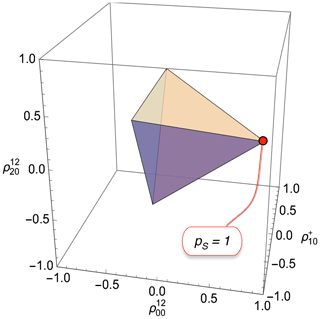Figure 3Physical bounds on the rank-0 polarization moment ${\mathit{\rho }}_{\mathrm{00}}^{\mathrm{12}}$, the rank-1 polarization moment ${\mathit{\rho }}_{\mathrm{10}}^{+}$, and the rank-2 polarization moment ${\mathit{\rho }}_{\mathrm{20}}^{\mathrm{12}}$ for spin-1/2 pairs. The shaded tetrahedron shows the physically accessible region. The vertices correspond to pure-state density operators for each of the four singlet or triplet states. The annotation shows the vertex for exclusive population of the singlet state (corresponding to pure parahydrogen in the case of H2 gas).

## 4.5 Spin-1/2 pairs

For spin-1/2 pairs, the symmetric μ=0 subspace is of dimension 4, spanned by the four symmetric spherical tensor operators given in Eq. (19). Since the polarization moment ${\mathit{\rho }}_{\mathrm{00}}^{\mathrm{0}}$ is fixed (Eq. 20), the symmetric part of the spin density operator may be described as a point with coordinates $\mathit{\left\{}{\mathit{\rho }}_{\mathrm{00}}^{\mathrm{12}},{\mathit{\rho }}_{\mathrm{10}}^{+},{\mathit{\rho }}_{\mathrm{20}}^{\mathrm{12}}\mathit{\right\}}$, given by its projections onto the three orthonormal spherical tensor operators $\mathit{\left\{}{\mathbb{T}}_{\mathrm{00}}^{\mathrm{12}},{\mathbb{T}}_{\mathrm{10}}^{+},{\mathbb{T}}_{\mathrm{20}}^{\mathrm{12}}\mathit{\right\}}$. The density operator may also include components that are antisymmetric with respect to exchange: these components lie outside this three-dimensional subspace and are not considered further here.

The physical bounds on the symmetrical polarization moments $\mathit{\left\{}{\mathit{\rho }}_{\mathrm{00}}^{\mathrm{12}},{\mathit{\rho }}_{\mathrm{10}}^{+},{\mathit{\rho }}_{\mathrm{20}}^{\mathrm{12}}\mathit{\right\}}$ are set by the following inequalities:

$\begin{array}{}\text{(32)}& \begin{array}{rl}\mathrm{0}\phantom{\rule{0.33em}{0ex}}\le & \frac{\mathrm{1}}{\mathrm{4}}\left(\mathrm{1}+\mathrm{2}\sqrt{\mathrm{3}}{\mathit{\rho }}_{\mathrm{00}}^{\mathrm{12}}\right)\phantom{\rule{0.33em}{0ex}}\le \phantom{\rule{0.33em}{0ex}}\mathrm{1}\\ \mathrm{0}\phantom{\rule{0.33em}{0ex}}\le & \frac{\mathrm{1}}{\mathrm{12}}\left(\mathrm{3}-\mathrm{2}\sqrt{\mathrm{3}}{\mathit{\rho }}_{\mathrm{00}}^{\mathrm{12}}+\mathrm{6}\sqrt{\mathrm{2}}{\mathit{\rho }}_{\mathrm{10}}^{+}+\mathrm{2}\sqrt{\mathrm{6}}{\mathit{\rho }}_{\mathrm{20}}^{\mathrm{12}}\right)\phantom{\rule{0.33em}{0ex}}\le \phantom{\rule{0.33em}{0ex}}\mathrm{1}\\ \mathrm{0}\phantom{\rule{0.33em}{0ex}}\le & \frac{\mathrm{1}}{\mathrm{12}}\left(\mathrm{3}-\mathrm{2}\sqrt{\mathrm{3}}{\mathit{\rho }}_{\mathrm{00}}^{\mathrm{12}}-\mathrm{4}\sqrt{\mathrm{6}}{\mathit{\rho }}_{\mathrm{20}}^{\mathrm{12}}\right)\phantom{\rule{0.33em}{0ex}}\le \phantom{\rule{0.33em}{0ex}}\mathrm{1}\\ \mathrm{0}\phantom{\rule{0.33em}{0ex}}\le & \frac{\mathrm{1}}{\mathrm{12}}\left(\mathrm{3}-\mathrm{2}\sqrt{\mathrm{3}}{\mathit{\rho }}_{\mathrm{00}}^{\mathrm{12}}-\mathrm{6}\sqrt{\mathrm{2}}{\mathit{\rho }}_{\mathrm{10}}^{+}+\mathrm{2}\sqrt{\mathrm{6}}{\mathit{\rho }}_{\mathrm{20}}^{\mathrm{12}}\right)\phantom{\rule{0.33em}{0ex}}\le \phantom{\rule{0.33em}{0ex}}\mathrm{1}\\ & \phantom{\rule{0.25em}{0ex}}\phantom{\rule{0.25em}{0ex}}\text{for}\phantom{\rule{0.25em}{0ex}}\text{spin-1/2}\phantom{\rule{0.25em}{0ex}}\text{pairs}.\end{array}\end{array}$

These inequalities constrain the polarization moments to the interior of the regular tetrahedron shown in Fig. 3. The vertices of the tetrahedron are at coordinates $\mathit{\left\{}{\mathit{\rho }}_{\mathrm{00}}^{\mathrm{12}},{\mathit{\rho }}_{\mathrm{10}}^{+},{\mathit{\rho }}_{\mathrm{20}}^{\mathrm{12}}\mathit{\right\}}$ given by

Each vertex corresponds to a pure-state density operator, in which the singlet state or one of the three triplet states is exclusively populated.

The highlighted point in Fig. 3 has coordinates $\mathit{\left\{}\frac{\mathrm{1}}{\mathrm{2}}{\mathrm{3}}^{\mathrm{1}/\mathrm{2}},\mathrm{0},\mathrm{0}\mathit{\right\}}$. From Eq. (21), this point corresponds to unit singlet polarization (pS=1) and hence a pure singlet density operator:

$\begin{array}{}\text{(34)}& \mathit{\rho }=\mathrm{|}{S}_{\mathrm{0}}〉〈{S}_{\mathrm{0}}\mathrm{|},\end{array}$

where the singlet state is given by (Levitt2019)

$\begin{array}{}\text{(35)}& \mathrm{|}{S}_{\mathrm{0}}〉=\frac{\mathrm{1}}{\sqrt{\mathrm{2}}}\left(\mathrm{|}\mathit{\alpha }\mathit{\beta }〉-\mathrm{|}\mathit{\beta }\mathit{\alpha }〉\right)\end{array}$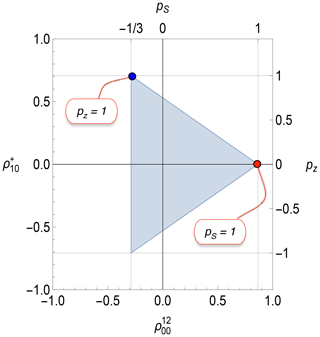Figure 4Physical bounds on the rank-0 polarization moment ${\mathit{\rho }}_{\mathrm{00}}^{\mathrm{12}}$ and the rank-1 polarization moment ${\mathit{\rho }}_{\mathrm{10}}^{+}$ for spin-1/2 pairs. The corresponding values of the z polarization pz and singlet polarization pS are also shown. The singlet polarization pS (top horizontal axis) and rank-0 polarization moment ${\mathit{\rho }}_{\mathrm{00}}^{\mathrm{12}}$ (lower horizontal axis) are related through Eq. (21). The shaded triangle shows the physically accessible region. The annotations show the vertices for exclusive population of the singlet state (red) and for maximal z polarization (blue). Note that complete z polarization is accompanied by singlet polarization of ${p}_{\mathrm{S}}=-\mathrm{1}/\mathrm{3}$.

A projection of the tetrahedral bound in Fig. 3 onto the $\mathit{\left\{}{\mathit{\rho }}_{\mathrm{00}}^{\mathrm{12}},{\mathit{\rho }}_{\mathrm{10}}^{+}\mathit{\right\}}$ plane is shown in Fig. 4. The corresponding values of the singlet polarization pS and z polarization pz are shown on the axes, with the conversion factors given in Eqs. (21) and (22). The red point in Fig. 4 shows that maximal singlet polarization is necessarily accompanied by zero z polarization. In the case that the spin-1/2 pair is composed of the two proton nuclei of H2, the red point corresponds to the spin density operator of pure parahydrogen (Farkas1935).

The blue point in Fig. 4 shows that maximal z polarization is necessarily accompanied by singlet polarization of ${p}_{\mathrm{S}}=-\mathrm{1}/\mathrm{3}$. This reflects the fact that maximal z polarization can only be achieved by depleting the singlet state at the expense of one of the triplet states. This fact may be exploited experimentally to generate hyperpolarized (negative) long-lived singlet order by the application of low-temperature dynamic nuclear polarization to spin-pair systems . Analogous phenomena are observed in more complex spin systems, such as methyl groups and deuterated moieties .

The physical bounds depicted in Figs. 3 and 4 are an intrinsic property of the spin-pair density operator and are completely independent of the spin Hamiltonian and its symmetry properties. Hence, these bounds apply to both magnetically equivalent and magnetically inequivalent spin-1/2 pairs. Nevertheless, since the spin Hamiltonian of magnetically inequivalent spin-1/2 pairs lacks exchange symmetry, it is also true that the density operator of magnetically inequivalent pairs readily accesses dimensions of Liouville space which are not exchange-symmetric and which are not included in these pictures. Hence, although Figs. 3 and 4 are equally valid for magnetically equivalent and inequivalent systems, there are additional dimensions which are not represented in these pictures and which are particularly relevant for the magnetically inequivalent case.

The geometry of the physical bounds is independent of the operator basis. Although a spherical tensor operator basis has been used in the discussion above, bounds of the same form are generated in any orthonormal operator basis, albeit with an overall rotation that depends on the relationship of the two bases. For example, if the ket–bra operator products |i〉〈j| are used as the basis of Liouville space, where $i,j\in \mathit{\left\{}\mathrm{1}\mathrm{\dots }{N}_{H}\mathit{\right\}}$, then the NH vertices of the bounding simplex are located at coordinates $\mathit{\left\{}\mathrm{1},\mathrm{0},\mathrm{0}\mathrm{\dots }\mathit{\right\}}$, $\mathit{\left\{}\mathrm{0},\mathrm{1},\mathrm{0},\mathrm{0}\mathrm{\dots }\mathit{\right\}}$, $\mathit{\left\{}\mathrm{0},\mathrm{0},\mathrm{1},\mathrm{0},\mathrm{\dots }\mathit{\right\}}$, etc., representing the pure-state density operators with exclusive population of a single Hilbert-space state.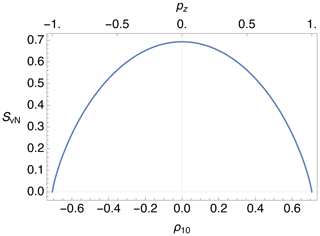Figure 5von Neumann entropy SvN plotted against the rank-1 polarization moment ρ10 and the z polarization pz for isolated spins-1/2, for the case of zero transverse polarizations ${p}_{x}={p}_{y}=\mathrm{0}$. The maximum value of SvN is ln 2≃0.693.

5 von Neumann entropy

Quantum statistical mechanics uses the von Neumann entropy (vNE) to describe the disorder in, or absence of information about, a quantum system . It is derived from the spin density operator as follows:

$\begin{array}{}\text{(36)}& {S}_{\mathrm{vN}}=-\text{Tr}\mathit{\left\{}\mathit{\rho }\mathrm{ln}\mathit{\rho }\mathit{\right\}}.\end{array}$

The vNE for a system in a pure quantum state is zero, while the vNE for a system with equal populations of NH quantum states, and no coherences, is given by SvN=ln NH.

## 5.1 Spins-1/2

The von Neumann entropy SvN is plotted against the rank-1 polarization moment ρ10 in Fig. 5, assuming that ρ1μ=0 for $\mathit{\mu }=±\mathrm{1}$. The corresponding value of the z polarization ${p}_{z}=\sqrt{\mathrm{2}}{\mathit{\rho }}_{\mathrm{10}}$ is shown on the top margin of the plot. The entropy goes to zero for complete z polarization in the positive or negative sense (${p}_{z}=±\mathrm{1}$) and attains the maximum value of SvN=ln 2 for zero polarization. The maximum entropy of ln 2 reflects the equal populations of the two Zeeman eigenstates and absence of coherences, for a completely saturated system.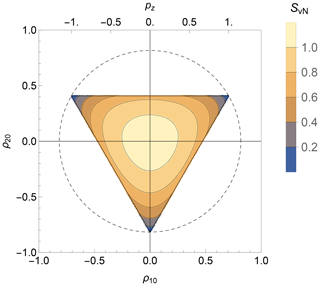Figure 6The von Neumann entropy SvN is shown as a contour plot against the rank-1 and rank-2 polarization moments for isolated spins-1, for the case of ρλμ=0 for μ≠0. Only the physical region is shown (see Fig. 1). The corresponding value of the z polarization ${p}_{z}={\mathrm{2}}^{\mathrm{1}/\mathrm{2}}{\mathit{\rho }}_{\mathrm{10}}$ is shown along the top edge. The maximum value of the von Neumann entropy, reached at the origin, is ln 3≃1.10.

## 5.2 Spins-1

For isolated spins-1, the von Neumann entropy is a function of the rank-1 and rank-2 polarization moments, assuming that all polarization moments ρλμ vanish for μ≠0. Figure 6 shows a contour plot of the von Neumann entropy against ρ10 and ρ20, assuming that all polarization moments with μ≠0 vanish. Only the physically allowed region is shown, delineated by the triangle, as in Fig. 1. The entropy goes to zero at the three vertices, which correspond to the pure-state density operators with 100 % population of a single state. The von Neumann entropy reaches the maximum value of ln 3 at the centre of the plot, corresponding to ${\mathit{\rho }}_{\mathrm{10}}={\mathit{\rho }}_{\mathrm{20}}=\mathrm{0}$. The value of ln 3 reflects the equal distribution of population over the three spin states.

## 5.3 Higher spins

The behaviour of the von Neumann entropy is readily anticipated for higher spin quantum numbers. The entropy vanishes at the (2I+1) vertices of the 2I-simplex which bounds the physical region. The entropy maximum of ln (2I+1) is reached at the origin of the space, which corresponds to equal populations for all of the 2I+1 spin states.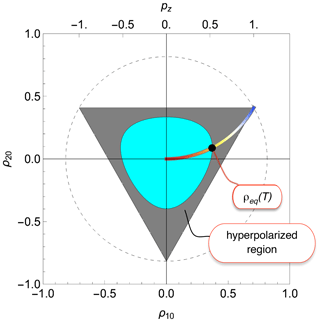Figure 7The coloured arc shows the set of thermal equilibrium density operators for spins-1 subjected to a dominant magnetic field. The spin temperature is indicated by colour, progressing from high (red) to low (blue). The black dot indicates the thermal equilibrium density operator at a particular temperature T. All points within the dark grey region represent hyperpolarized states of the spin-1 ensemble (density operators) at temperature T.

6 Hyperpolarization

## 6.1 Thermal equilibrium

Thermal equilibrium with the environment at temperature T is reached when the density operator adopts the following form:

$\begin{array}{}\text{(37)}& {\mathit{\rho }}_{\mathrm{eq}}\left(T\right)=\frac{\mathrm{exp}\mathit{\left\{}-{H}_{\mathrm{coh}}/{k}_{B}T\mathit{\right\}}}{\text{Tr}\mathit{\left\{}\mathrm{exp}\mathit{\left\{}-{H}_{\mathrm{coh}}/{k}_{B}T\mathit{\right\}}\mathit{\right\}}},\end{array}$

where Hcoh is the coherent part of the spin Hamiltonian (excluding all fluctuating terms which drive dissipation). Equation (37) describes a Boltzmann distribution of spin-state populations under the coherent Hamiltonian Hcoh.

In many cases, the coherent Hamiltonian is dominated by the Zeeman interaction with the main magnetic field, Hcohω0Iz, where the Larmor frequency is ${\mathit{\omega }}^{\mathrm{0}}=-\mathit{\gamma }{B}^{\mathrm{0}}$, and B0 is the magnetic field. In this case the thermal equilibrium density operator is given by

$\begin{array}{}\text{(38)}& {\mathit{\rho }}_{\mathrm{eq}}\simeq \frac{\mathrm{exp}\mathit{\left\{}\mathit{\beta }{I}_{z}\mathit{\right\}}}{\text{Tr}\mathit{\left\{}\mathrm{exp}\mathit{\left\{}\mathit{\beta }{I}_{z}\mathit{\right\}}\mathit{\right\}}},\end{array}$

where the normalized inverse temperature is $\mathit{\beta }=-{\mathit{\omega }}^{\mathrm{0}}/{k}_{B}T$. Since Eq. (38) is non-linear in Iz, the thermal equilibrium density operator contains high-rank polarization moments in thermal equilibrium.

The coloured arc in Fig. 7 shows the set of thermal equilibrium density operators for a dominant Zeeman interaction (Eq. 38), over a range of spin temperatures. Blue denotes a low spin temperature (β→∞), while red denotes a high spin temperature (β→0). Note the increase in the rank-2 polarization moment ρ20 at low spin temperatures.

## 6.2 A criterion of hyperpolarization

The von Neumann entropy in thermal equilibrium at temperature T is given by

$\begin{array}{}\text{(39)}& {S}_{\mathrm{vN}}^{\mathrm{eq}}\left(T\right)=-\text{Tr}\mathit{\left\{}{\mathit{\rho }}_{\mathrm{eq}}\left(T\right)\mathrm{ln}{\mathit{\rho }}_{\mathrm{eq}}\left(T\right)\mathit{\right\}},\end{array}$

where the thermal equilibrium density operator is given by Eq. (37). We propose the following criterion of hyperpolarization:

where T is the temperature of the environment. Note that this definition of hyperpolarization makes no explicit mention of population differences or the existence of a net magnetic moment in a certain direction.

The criterion in Eq. (40) identifies a region of Liouville space which is occupied by hyperpolarized states. For example, since the black dot in Fig. 7 indicates the thermal equilibrium density operator for spins I=1 at temperature T, the contour line ${S}_{\mathrm{vN}}={S}_{\mathrm{vN}}^{\mathrm{eq}}\left(T\right)$ delineates the region of hyperpolarization at temperature T. All density operators which are inside the dark grey region represent physically realizable hyperpolarized states of the spin ensemble.

Being inside the dark grey region is a sufficient but not necessary criterion of hyperpolarization. Points outside the dark grey region but within the pale blue region might also represent hyperpolarized states, in the case that polarization moments which are not represented in the diagram, i.e. ρλμ with $\mathit{\left\{}\mathit{\lambda },\mathit{\mu }\mathit{\right\}}\ne \mathit{\left\{}\mathrm{1},\mathrm{0}\mathit{\right\}}$ and {2,0}, are sufficiently large.

The criterion in Eq. (40) is readily applied to higher spin systems, including coupled spin systems. Under this definition, parahydrogen is hyperpolarized, since the corresponding density operator has a von Neumann entropy of zero, which is lower than that of any thermal equilibrium state at finite temperature, even though pure parahydrogen possesses no magnetic moment or net angular momentum in a given direction.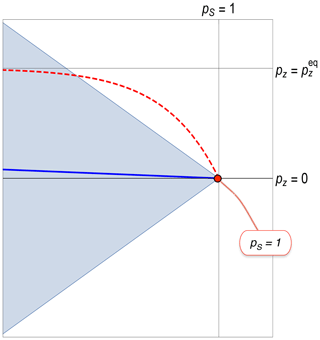Figure 8Non-equilibrium spin dynamics for an ensemble of spin-1/2 pairs, with TST1, where TS is the rate constant for the decay of singlet order, and T1 is the rate constant for the equilibration of z polarization. The plot shows an expanded view of Fig. 4 in the vicinity of the red dot, which represents an initial state of 100 % singlet polarization. Dashed red line: trajectory predicted by the inhomogeneous master equation. Solid blue line: trajectory predicted by the Lindbladian master equation. Both trajectories eventually lead to the same thermal equilibrium state, represented by a point with coordinates $\mathit{\left\{}\mathrm{0},{{p}_{z}}^{\mathrm{eq}}\mathit{\right\}}$, which is well beyond the left-hand edge of the plotted region and is not shown.

7 Non-equilibrium spin dynamics

The dynamics of the spin density operator is governed by a differential equation called the master equation which takes into account coherent influences on the spin system (such as external magnetic fields and non-fluctuating components of the spin interactions) as well as relaxation effects. Various forms of the master equation have been proposed. The most widely used form is called the inhomogeneous master equation, which has the following form:

$\begin{array}{}\text{(41)}& \frac{\mathrm{d}}{\mathrm{d}t}\mathit{\rho }=-i{\stackrel{\mathrm{^}}{H}}_{\mathrm{coh}}\mathit{\rho }\left(t\right)+\stackrel{\mathrm{^}}{\mathrm{\Gamma }}\left(\mathit{\rho }\left(t\right)-{\mathit{\rho }}_{\mathrm{eq}}\right),\end{array}$

where ${\stackrel{\mathrm{^}}{H}}_{\mathrm{coh}}$ is the commutation superoperator of the coherent Hamiltonian, and $\stackrel{\mathrm{^}}{\mathrm{\Gamma }}$ is the relaxation superoperator . The equilibrium spin density operator ρeq is given by Eq. (37).

The inhomogeneous master Eq. (41) is valid for high-entropy states which are close to equilibrium and is a standard component of NMR theory . However, in a previous paper , we showed that Eq. (41) loses validity for low-entropy states and may, in some cases, lead to non-physical predictions. We proposed a Lindbladian master equation, which has a wider range of validity.

This point is reinforced by Fig. 8, which compares the predictions of the inhomogeneous and Lindbladian master equations when applied to spin-1/2 pairs in a low-entropy state of pure singlet polarization. The initial state of pure singlet order is shown by the red dot. The plot shows an expanded view of Liouville space, in the vicinity of the initial condition. The physical bounds of Liouville space are indicated by the blue triangle, as in Fig. 4.

The red dashed line shows the trajectory predicted by the IME, in the case that TST1, where TS is the relaxation time constant for singlet order (Levitt2019) and T1 is the relaxation time constant for z polarization. Since T1 is relatively short, the z polarization rapidly assumes its thermal equilibrium value ${\mathit{\rho }}_{z}^{\mathrm{eq}}$, which is finite in the presence of a strong magnetic field. However, as shown in Fig. 8, this leads the density operator into a forbidden region outside the physical boundary of Liouville space. This proves that the inhomogeneous master equation must be invalid in this regime.

The predicted trajectory of the Lindbladian master equation, as described in , is shown by the blue line. This uneventful trajectory always stays well within the physical boundary of Liouville space.

8 Conclusions

This article has been an exploration of the geometry and physical boundary of Liouville space, the home territory of all spin density operators. In the past, most NMR experiments have only explored a tiny region of this space, very close to the origin (except for the fixed projection onto the unity operator). However, NMR experiments are increasingly performed on highly non-equilibrium spin states, which are sometimes located on or near the physical Liouville space boundary. We hope that this article is useful as a partial guide for wanderers in this region.

The word “partial” is used deliberately. So far, we have concentrated on the aspects of Liouville space which concern populations, and in the case of spin-1/2 pairs, on operators that are exchange-symmetric. The map still needs to be completed by delineating the physical bounds on coherences and on operators for multiple-spin systems, including those that are not exchange-symmetric. There has already been significant progress in that direction .

The physical bounds discussed in this article should not be confused with the bounds on the unitary transformations of density operators , which may also be represented by convex polytopes . The relationship between these different geometric bounds is another topic for future research.

Code availability

The software code for the graphics shown in this paper is available from the authors on reasonable request.

Data availability

Author contributions

MHL and CB contributed theory. MHL wrote the manuscript.

Competing interests

The authors declare that they have no conflict of interest.

Special issue statement

Acknowledgements

This paper is dedicated to the memory of Kostya Ivanov: our collaboration was fruitful and inspiring, but too brief. We also thank James Eills, Jean-Nicolas Dumez, Thomas Schulte-Herbrüggen, Dima Budker, and Phil Kuchel for discussions. Malcolm H. Levitt would like to express his heartfelt thanks to Geoffrey Bodenhausen for many years of friendship, guidance, support, encouragement, illuminating insights, and furious arguments.

Financial support

This research has been supported by the Research Councils UK (grant no. EP/P009980/1) and the H2020 European Research Council (grant no. 786707-FunMagResBeacons).

Review statement

This paper was edited by Daniel Abergel and reviewed by two anonymous referees.

References

Abragam, A.: The Principles of Nuclear Magnetism, Clarendon Press, Oxford, ISBN 0-19-852014-X, 1961. a, b

Adams, R. W., Aguilar, J. A., Atkinson, K. D., Cowley, M. J., Elliott, P. I. P., Duckett, S. B., Green, G. G. R., Khazal, I. G., Lopez-Serrano, J., and Williamson, D. C.: Reversible Interactions with Para-Hydrogen Enhance NMR Sensitivity by Polarization Transfer, Science, 323, 1708–1711, 2009. a

Aghelnejad, B., Marhabaie, S., Baudin, M., Bodenhausen, G., and Carnevale, D.: Spin Thermometry: A Straightforward Measure of Millikelvin Deuterium Spin Temperatures Achieved by Dynamic Nuclear Polarization, J. Phys. Chem. Lett., 11, 3219–3225, 2020. a

Ahuja, P., Sarkar, R., Vasos, P. R., and Bodenhausen, G.: Diffusion Coefficients of Biomolecules Using Long-Lived Spin States, J. Am. Chem. Soc., 131, 7498–7499, 2009. a

Ardenkjaer-Larsen, J. H., Fridlund, B., Gram, A., Hansson, G., Hansson, L., Lerche, M. H., Servin, R., Thaning, M., and Golman, K.: Increase in Signal-to-Noise Ratio of > 10,000 Times in Liquid-State NMR, P. Natl. Acad. Sci. USA, 100, 10158–10163, 2003. a

Auzinsh, M., Budker, D., and Rochester, S.: Optically Polarized Atoms: Understanding Light-Atom Interactions, Oxford University Press, Oxford, New York, ISBN 978-0-19-870502-4, 2014. a, b

Bain, A. D.: Modulation of NMR Spin Echoes in Coupled Systems, Chem. Phys. Lett., 57, 281–284, 1978. a, b

Bain, A. D.: A Superspin Analysis of Two-Dimensional FT NMR Experiments, J. Magn. Reson., 39, 335–342, 1980a. a, b

Bain, A. D.: Superspin in NMR: Application to the ABX System, J. Magn. Reson., 37, 209–216, 1980b. a, b

Bain, A. D.: Coherence Levels and Coherence Pathways in NMR. A Simple Way to Design Phase Cycling Procedures, J. Magn. Reson., 56, 418–427, 1984. a

Banwell, C. N. and Primas, H.: On the Analysis of High-Resolution Nuclear Magnetic Resonance Spectra, Mol. Phys., 6, 225–256, 1963. a

Batchelder, L. S.: Deuterium NMR in Solids, in: eMagRes, Wiley, Chichester, UK, ISBN 978-0-470-03459-0, 2007. a

Bengs, C. and Levitt, M. H.: A Master Equation for Spin Systems Far from Equilibrium, J. Magn. Reson., 310, 106645, https://doi.org/10.1016/j.jmr.2019.106645, 2020. a, b, c

Bengtsson, I. and Zyczkowski, K.: Geometry of Quantum States: An Introduction to Quantum Entanglement, Cambridge University Press, Cambridge, ISBN 978-0-511-53504-8, 2006. a

Bodenhausen, G., Kogler, H., and Ernst, R. R.: Selection of Coherence-Transfer Pathways in NMR Pulse Experiments, J. Magn. Reson., 58, 370–388, 1984. a

Bornet, A., Ji, X., Mammoli, D., Vuichoud, B., Milani, J., Bodenhausen, G., and Jannin, S.: Long-Lived States of Magnetically Equivalent Spins Populated by Dissolution-DNP and Revealed by Enzymatic Reactions, Chem. Eur. J., 20, 17113–17118, 2014. a

Bowden, G. J. and Hutchison, W. D.: Tensor Operator Formalism for Multiple-Quantum NMR. 3. Spin-1 Nuclei with an Asymmetry Term in the Quadrupole Hamiltonian, J. Magn. Reson., 70, 361–367, 1986a. a, b

Bowden, G. J. and Hutchison, W. D.: Tensor Operator Formalism for Multiple-Quantum NMR. 1. Spin-1 Nuclei, J. Magn. Reson., 67, 403–414, 1986b. a, b

Bowden, G. J. and Hutchison, W. D.: Tensor Operator Formalism for Multiple-Quantum NMR. 4. Spin-32 Nuclei with an Asymmetry Term in the Quadrupole Hamiltonian, J. Magn. Reson., 72, 61–74, 1987. a

Bowden, G. J., Hutchison, W. D., and Khachan, J.: Tensor Operator Formalism for Multiple-Quantum NMR. 2. Spins 3 2, 2, and 5 2 and General I, J. Magn. Reson., 67, 415–437, 1986. a, b

Bowden, G. J., Martin, J. P. D., and Separovic, F.: Tensorial Sets for Coupled Pairs of Spin-1/2 Nuclei, Mol. Phys., 70, 581–603, 1990. a, b, c

Bowers, C. R. and Weitekamp, D. P.: Parahydrogen and Synthesis Allow Dramatically Enhanced Nuclear Alignment, J. Am. Chem. Soc., 109, 5541–5542, 1987. a

Breuer, H.-P. and Petruccione, F.: The Theory of Open Quantum Systems, Oxford University Press, Oxford [England]; New York, ISBN 978-0-19-921390-0, 2010. a

Budker, D., Gawlik, W., Kimball, D. F., Rochester, S. M., Yashchuk, V. V., and Weis, A.: Resonant Nonlinear Magneto-Optical Effects in Atoms, Rev. Mod. Phys., 74, 1153–1201, 2002. a

Byrd, M. S. and Khaneja, N.: Characterization of the Positivity of the Density Matrix in Terms of the Coherence Vector Representation, Phys. Rev. A, 68, 062322, https://doi.org/10.1103/PhysRevA.68.062322, 2003. a

Carravetta, M. and Levitt, M. H.: Long-Lived Nuclear Spin States in High-Field Solution NMR, J. Am. Chem. Soc., 126, 6228–6229, 2004. a

Carravetta, M., Johannessen, O. G., and Levitt, M. H.: Beyond the T1 Limit: Singlet Nuclear Spin States in Low Magnetic Fields, Phys. Rev. Lett., 92, 153003, https://doi.org/10.1103/PhysRevLett.92.153003, 2004. a

Carravetta, M., Danquigny, A., Mamone, S., Cuda, F., Johannessen, O. G., Heinmaa, I., Panesar, K., Stern, R., Grossel, M. C., Horsewill, A. J., Samoson, A., Murata, M., Murata, Y., Komatsu, K., and Levitt, M. H.: Solid-State NMR of Endohedral Hydrogen-Fullerene Complexes, Phys. Chem. Chem. Phys., 9, 4879–4894, 2007. a

Cavadini, S., Dittmer, J., Antonijevic, S., and Bodenhausen, G.: Slow Diffusion by Singlet State NMR Spectroscopy, J. Am. Chem. Soc., 127, 15744–15748, 2005. a

Coxeter, H. S. M.: Regular Polytopes, Macmillan, New York, 2nd edn., ISBN 0-486-14158-6, 1963. a, b, c

Dumez, J.-N.: Perspective on Long-Lived Nuclear Spin States, Mol. Phys., 118, e1644382, https://doi.org/10.1080/00268976.2019.1644382, 2019. a

Dumez, J.-N., Håkansson, P., Mamone, S., Meier, B., Stevanato, G., Hill-Cousins, J. T., Roy, S. S., Brown, R. C. D., Pileio, G., and Levitt, M. H.: Theory of Long-Lived Nuclear Spin States in Methyl Groups and Quantum-Rotor Induced Polarisation, J. Chem. Phys., 142, 044506, https://doi.org/10.1063/1.4906273, 2015. a

Dumez, J.-N., Vuichoud, B., Mammoli, D., Bornet, A., Pinon, A. C., Stevanato, G., Meier, B., Bodenhausen, G., Jannin, S., and Levitt, M. H.: Dynamic Nuclear Polarization of Long-Lived Nuclear Spin States in Methyl Groups, J. Phys. Chem. Lett., 8, 3549–3555, 2017. a

Ernst, R. R., Bodenhausen, G., and Wokaun, A.: Principles of Nuclear Magnetic Resonance in One and Two Dimensions, Clarendon Press, Oxford, ISBN 978-0-19-855647-3, 1987. a, b, c, d, e, f, g

Farkas, A.: Orthohydrogen, Parahydrogen and Heavy Hydrogen, Cambridge University Press, Cambridge, OCLC: 622966476, 1935. a

Garon, A., Zeier, R., and Glaser, S. J.: Visualizing Operators of Coupled Spin Systems, Phys. Rev. A, 91, 042122, https://doi.org/10.1103/PhysRevA.91.042122, 2015. a, b, c

Goyal, S. K., Simon, B. N., Singh, R., and Simon, S.: Geometry of the Generalized Bloch Sphere for Qutrits, J. Phys. A, 49, 165203, https://doi.org/10.1088/1751-8113/49/16/165203, 2016. a, b, c

Griffin, R. G. and Prisner, T. F.: High Field Dynamic Nuclear Polarization – the renaissance, Phys. Chem. Chem. Phys., 12, 5737–5740, 2010. a

Icker, M. and Berger, S.: Unexpected Multiplet Patterns Induced by the Haupt-Effect, J. Magn. Reson., 219, 1–3, 2012. a

Jaccard, G., Wimperis, S., and Bodenhausen, G.: Multiple-quantum NMR Spectroscopy of S=3/2 Spins in Isotropic Phase: A New Probe for Multiexponential Relaxation, J. Chem. Phys., 85, 6282–6293, 1986. a

Jannin, S., Bornet, A., Melzi, R., and Bodenhausen, G.: High Field Dynamic Nuclear Polarization at 6.7T: Carbon-13 Polarization above 70 % within 20 min, Chem. Phys. Lett., 549, 99–102, 2012. a

Jeener, J.: Superoperators in Magnetic Resonance, edited by: Waugh, J. S., Advances in Magnetic and Optical Resonance, 10, 1–51, 1982. a

Kastler, A.: Optical Methods of Atomic Orientation and of Magnetic Resonance, J. Opt. Soc. Am. (JOSA), 47, 460–465, 1957. a

Kimura, G. and Kossakowski, A.: The Bloch-Vector Space for N-Level Systems: The Spherical-Coordinate Point of View, Open Syst. Inf. Dyn., 12, 207–229, 2005. a, b

Kress, T., Walrant, A., Bodenhausen, G., and Kurzbach, D.: Long-Lived States in Hyperpolarized Deuterated Methyl Groups Reveal Weak Binding of Small Molecules to Proteins, J. Phys. Chem. Lett., 10, 1523–1529, 2019. a

Levitt, M. H.: Thermodynamics of Hartmann-Hahn Cross-Polarization, in: Pulsed Magnetic Resonance: NMR, ESR and Optics. A Recognition of E. L. Hahn, edited by: Bagguley, D. M. S., Oxford University Press, Oxford, OCLC: 1176441427, 1992a. a, b

Levitt, M. H.: Unitary Evolution, Liouville Space and Local Spin Thermodynamics, J. Magn. Reson., 99, 1–17, 1992b. a, b

Levitt, M. H.: An Orientational Sampling Scheme for Magnetic Resonance Based on a Four-Dimensional Polytope, in: Future Directions of NMR, edited by Khetrapal, C. L., Kumar, A., and Ramanathan, K. V., Springer India, New Delhi, India, 231–237, ISBN 978-81-8489-588-9, 2010. a

Levitt, M. H.: Symmetry Constraints on Spin Dynamics: Application to Hyperpolarized NMR, J. Magn. Reson., 262, 91–99, 2016. a

Levitt, M. H.: Long Live the Singlet State!, J. Magn. Reson., 306, 69–74, 2019. a, b, c

Mammoli, D., Vuichoud, B., Bornet, A., Milani, J., Dumez, J.-N., Jannin, S., and Bodenhausen, G.: Hyperpolarized Para-Ethanol, J. Phys. Chem. B, 119, 4048–4052, 2015. a

Mamone, S., Pileio, G., and Levitt, M. H.: Orientational Sampling Schemes Based on Four Dimensional Polytopes, Symmetry, 2, 1423–1449, 2010. a

Mehring, M.: High Resolution NMR Spectroscopy in Solids, NMR Basic Principles and Progress, Springer-Verlag, Berlin Heidelberg, ISBN 978-3-642-96334-6, 1976. a

Meier, B., Dumez, J.-N., Stevanato, G., Hill-Cousins, J. T., Roy, S. S., Håkansson, P., Mamone, S., Brown, R. C. D., Pileio, G., and Levitt, M. H.: Long-Lived Nuclear Spin States in Methyl Groups and Quantum-Rotor-Induced Polarization, J. Am. Chem. Soc., 135, 18746–18749, 2013. a

Navon, G., Song, Y.-Q., Rõõm, T., Appelt, S., Taylor, R. E., and Pines, A.: Enhancement of Solution NMR and MRI with Laser-Polarized Xenon, Science, 271, 1848–1851, 1996. a

Nielsen, N. C. and Sørensen, O. W.: Conditional Bounds on Polarization Transfer, J. Magn. Reson. Ser. A, 114, 24–31, 1995. a

Pell, A. J.: A Method to Calculate the NMR Spectra of Paramagnetic Species Using Thermalized Electronic Relaxation, J. Magn. Reson., 326, 106939, https://doi.org/10.1016/j.jmr.2021.106939, 2021. a

Philp, D. J. and Kuchel, P. W.: A Way of Visualizing NMR Experiments on Quadrupolar Nuclei, Concept. Magnetic Res. Part A, 25A, 40–52, 2005. a, b

Pileio, G. and Levitt, M. H.: Isotropic Filtering Using Polyhedral Phase Cycles: Application to Singlet State NMR, J. Magn. Reson., 191, 148–155, 2008. a

Redfield, A. G.: The Theory of Relaxation Processes, in: Advances in Magnetic and Optical Resonance, edited by: Waugh, J. S., Academic Press, Advances in Magnetic Resonance, 1, 1–32, ISBN 978-1-4832-3114-3, 1965. a, b

Rodin, B. A., Bengs, C., Kiryutin, A. S., Sheberstov, K. F., Brown, L. J., Brown, R. C. D., Yurkovskaya, A. V., Ivanov, K. L., and Levitt, M. H.: Algorithmic Cooling of Nuclear Spins Using Long-Lived Singlet Order, J. Chem. Phys., 152, 164201, https://doi.org/10.1063/5.0006742, 2020. a, b

Sanctuary, B. C.: Multipole Operators for an Arbitrary Number of Spins, J. Chem. Phys., 64, 4352–4361, 1976. a, b, c

Sanctuary, B. C.: Magnetic Multipoles in Time Dependent Fields, J. Chem. Phys., 73, 1048–1053, 1980. a, b, c

Sanctuary, B. C. and Temme, F. P.: Multipole N.M.R.: XIII. Multispin Interactions and Symmetry in Liouville Space, Mol. Phys., 55, 1049–1062, 1985a. a, b, c

Sanctuary, B. C. and Temme, F. P.: Multipole N.M.R., Molecular Physics, 55, 1049–1062, 1985b. a, b, c

Sarkar, R., Ahuja, P., Moskau, D., Vasos, P. R., and Bodenhausen, G.: Extending the Scope of Singlet-State Spectroscopy, ChemPhysChem, 8, 2652–2656, 2007a. a

Sarkar, R., Vasos, P. R., and Bodenhausen, G.: Singlet-State Exchange NMR Spectroscopy for the Study of Very Slow Dynamic Processes, J. Am. Chem. Soc., 129, 328–334, 2007b. a

Sørensen, O. W.: A Universal Bound on Spin Dynamics, J. Magn. Reson., 86, 435–440, 1990. a

Sørensen, O. W., Eich, G. W., Levitt, M. H., Bodenhausen, G., and Ernst, R. R.: Product Operator Formalism for the Description of NMR Pulse Experiments, Prog. Nucl. Mag. Res. Sp., 16, 163–192, 1984.  a, b

Spiess, H. W.: Rotation of Molecules and Nuclear Spin Relaxation, in: Dynamic NMR Spectroscopy, edited by: Steigel, A. and Spiess, H. W., Springer Berlin Heidelberg, NMR Basic Principles and Progress/Grundlagen Und Fortschritte, 15, 55–214, 1978. a

Suzuki, M. and Kubo, R.: Theoretical Calculation of NMR Spectral Line Shapes, Mol. Phys., 7, 201–209, 1964. a

Szymański, K., Weis, S., and Życzkowski, K.: Classification of Joint Numerical Ranges of Three Hermitian Matrices of Size Three, Linear Algebra Appl., 545, 148–173, 2018. a, b

Tayler, M. C. D., Marco-Rius, I., Kettunen, M. I., Brindle, K. M., Levitt, M. H., and Pileio, G.: Direct Enhancement of Nuclear Singlet Order by Dynamic Nuclear Polarization, J. Am. Chem. Soc., 134, 7668–7671, 2012. a

van Beek, J. D., Carravetta, M., Antonioli, G. C., and Levitt, M. H.: Spherical Tensor Analysis of Nuclear Magnetic Resonance Signals, J. Chem. Phys., 122, 244510, https://doi.org/10.1063/1.1943947, 2005. a

Varshalovich, D. A., Moskalev, A. N., and Kheronskii, V. K.: Quantum Theory of Angular Momentum, World Scientific, Singapore, ISBN 978-9971-5-0107-5, 1988. a